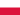Marginal Revenue and Marginal CostThe New Alison App has just launched

All Modules View all topics Close topics
Module 1: Understanding Fixed, Variable and Marginal Costs in Economics

# Marginal Revenue and Marginal Cost

• Study Reminders

We'll email you at these times to remind you to study

You can set up to 7 reminders per week

#### You're all set

We'll email you at these times to remind you to study

Monday

Tuesday

Wednesday

Thursday

Friday

Saturday

Sunday

• Study Notes
• Discuss This TopicKaram A. 0 0 here i would like to make a relation ship between Marginal Revenue and Marginal Cost,,, The marginal cost of production measures the change in total cost of a good that arises from producing one additional unit of that good. The marginal cost is calculated by dividing the change in the total cost by the change in quantity. Using calculus, the marginal cost is calculated by taking the first derivative of the total cost function with respect to the quantity: MC = dTC/dQ. For example, the total cost of producing 100 units of a good is \$200. The total cost of producing 101 units is \$204. The average cost of producing 100 units is \$2, or \$200/100; however, the marginal cost for producing the 101st unit is \$4, or (\$204 - \$200)/(101-100). The marginal revenue measures the change in the revenue that arises when one additional unit of a product is sold. The marginal revenue is calculated by dividing the change in the total revenue by the change in the quantity. In calculus terms, the marginal revenue is the first derivative of the total revenue function with respect to the quantity: MR = dTR/dQ. For example, suppose the price of a product is \$10 and a company produces 20 units per day. The total revenue is calculated by multiplying the price by the quantity produced. In this case, the total revenue is \$200, or \$10*20. The total revenue from producing 21 units is \$205. The marginal revenue is calculated as \$5, or (\$205 - \$200)/(21-20). When marginal revenue and the marginal cost of production is equal, profit is maximized at that level of output and price. In terms of calculus, the relationship is stated as: dTR/dQ = dTC/dQ When marginal revenue is less than the marginal cost of production, a company is producing too much and should decrease its quantity supplied until marginal revenue equals the marginal cost of production. When the marginal revenue is greater than the marginal cost, the firm is not producing enough goods and should increase its output until profit is maximized. Read more: How is marginal revenue related to the marginal cost of production? | Investopedia http://www.investopedia.com/ask/answers/041315/how-marginal-revenue-related-marginal-cost-production.asp#ixzz3vHDSNM4E Follow us: Investopedia on Facebook
• Text Version